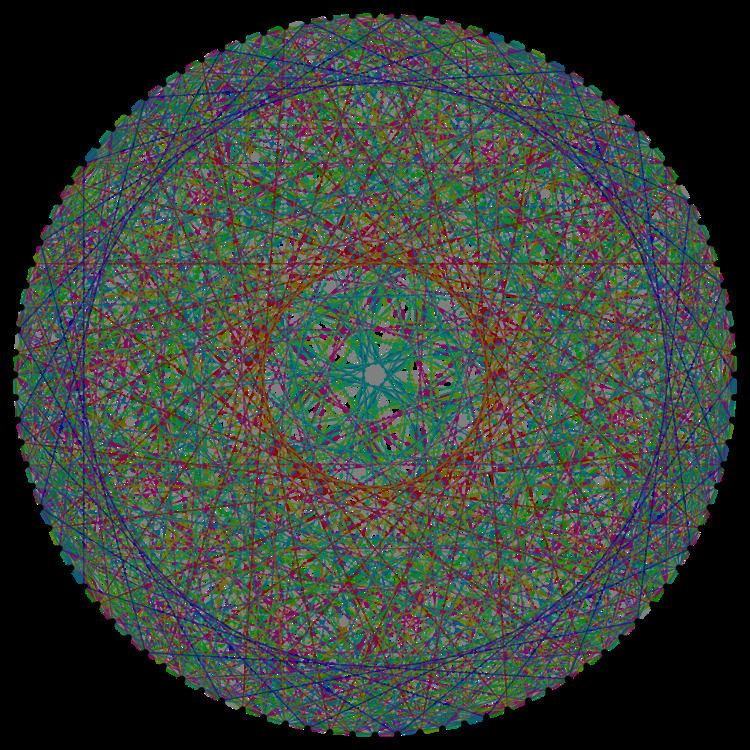# Higman–Sims graph

Updated on
Covid-19
 Vertices  100Radius  2Girth  4 Edges  1100Diameter  2Named after  Donald G. Higman Charles C. Sims

In mathematical graph theory, the Higman–Sims graph is a 22-regular undirected graph with 100 vertices and 1100 edges. It is the unique strongly regular graph with 100 vertices and valency 22, where no neighboring pair of vertices share a common neighbor and each non-neighboring pair of vertices share six common neighbors. It was first constructed by Mesner (1956) and rediscovered in 1968 by Donald G. Higman and Charles C. Sims as a way to define the Higman–Sims group, and that group is a subgroup of index two in the group of automorphisms of the Higman–Sims graph.

## Contents

Construction begins with the M22 graph, whose 77 vertices are the blocks of the S(3,6,22) Steiner system W22. Adjacent vertices are defined to be disjoint blocks. This graph is strongly regular; any vertex has 16 neighbors, any 2 adjacent vertices have no common neighbors, and any 2 non-adjacent vertices have 4 common neighbors. This graph has M22:2 as its automorphism group, M22 being a Mathieu group.

The Higman–Sims graph is then formed by appending the 22 points of W22 and a 100th vertex C. The neighbors of C are defined to be those 22 points. A point adjacent to a block is defined to be one that is included.

A Higman–Sims graph can be partitioned into two copies of the Hoffman–Singleton graph in 352 ways.

## Algebraic properties

The automorphism group of the Higman–Sims graph is a group of order 88,704,000 isomorphic to the semidirect product of the Higman–Sims group of order 44,352,000 with the cyclic group of order 2. It has automorphisms that take any edge to any other edge, making the Higman–Sims graph an edge-transitive graph.

The characteristic polynomial of the Higman–Sims graph is (x − 22)(x − 2)77(x + 8)22. Therefore the Higman–Sims graph is an integral graph: its spectrum consists entirely of integers. It is also the only graph with this characteristic polynomial, making it a graph determined by its spectrum.

## Inside the Leech lattice

The Higman–Sims graph naturally occurs inside the Leech lattice: if X, Y and Z are three points in the Leech lattice such that the distances XY, XZ and YZ are 2 , 6 , 6 respectively, then there are exactly 100 Leech lattice points T such that all the distances XT, YT and ZT are equal to 2, and if we connect two such points T and T′ when the distance between them is 6 , the resulting graph is isomorphic to the Higman–Sims graph. Furthermore, the set of all automorphisms of the Leech lattice (that is, Euclidean congruences fixing it) which fix each of X, Y and Z is the Higman–Sims group (if we allow exchanging X and Y, the order 2 extension of all graph automorphisms is obtained). This shows that the Higman–Sims group occurs inside the Conway groups Co2 (with its order 2 extension) and Co3, and consequently also Co1.

## References

Similar Topics
The Man from Texas
Paul Bagshaw
Georges Chometon
Topics